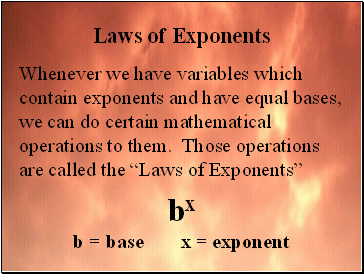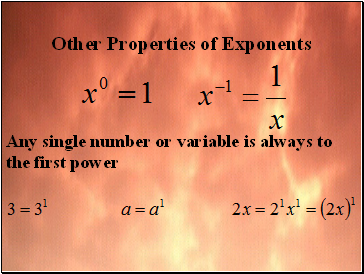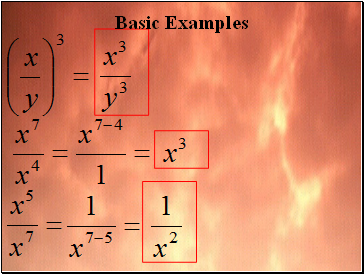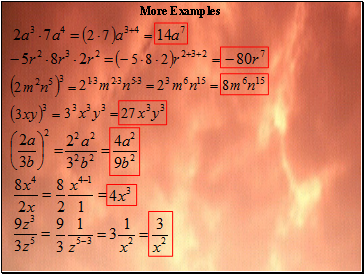# Laws of exponentsPage 1

#### WATCH ALL SLIDES

Slide 1## Laws of Exponents

Whenever we have variables which contain exponents and have equal bases, we can do certain mathematical operations to them. Those operations are called the “Laws of Exponents”

bx

b = base x = exponent

Slide 2Laws of Exponents

Slide 3## Other Properties of Exponents

Any single number or variable is always to the first power

Slide 4## Basic Examples

Slide 5Basic Examples

Slide 6More Examples

Slide 7More Examples